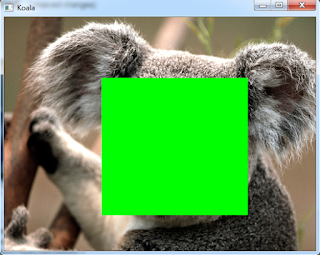### How to Draw Rectangle on An Image using OpenCV in Python

OpenCV (Open Source Computer Vision) is an open source computer vision library. To work with computer vision problems OpenCV is very helpful. We can perform many tasks using OpenCV like Recognition, Motion Analysis, Scene Reconstruction, image restoration.

We use  `cv2.rectangle()`  to draw rectangle on an image. This method is used to draw bounding boxes of the objects in object detection task.

• Python
• OpenCV

#### Install OpenCV

If you have not already installed OpenCV on you system, you can install it using pip command as written below.

`pip install opencv-python`

#### Syntax

The syntax to draw rectangle on an image is as below.

`cv2.rectangle(image, start_point, end_point, color, thickness)`

`Parameters: image: image on that the rectangle to drawnstart_point:(width_min, height_min)= (xmin, ymin):start coordinate of rectangle. It is top left point on the image.end_point: (width_max, height_max)= (xmax, yax):end coordinate of rectangle. It’s bottom right point on the image.color: (B,G,R)-  Color of the linethickness:  Thickness of the line`

#### Steps

The steps to draw rectangle on an image is as follows
2. define starting and ending point of the rectangle
3. Define the color of the line of the rectangle
4. Define the thickness of the line of the rectangle
5. Draw the rectangle
6. Display the image with drawn rectangle

#### Example 1.

Let's write complete Python 3 program for drawing rectangle on the image.

`# Python 3 program to draw rectangle on an image# import cv2import cv2# Read an image img = cv2.imread('Koala.jpg')# Start coordinate (xmin, ymin), it represents the top left corner of rectanglestart_point = (320, 200)# End coordinate (xmax, ymax), it represents the bottom right corner of rectangleend_point = (800, 650)# Green color in BGRcolor = (0, 255, 0)# Line thickness of 4 pxthickness = 4# Using cv2.rectangle() # Draw a rectangle with green line of thickness of 4 pximg = cv2.rectangle(img, start_point, end_point, color, thickness)# Display the imagecv2.imshow("Koala", img)cv2.waitKey(0) cv2.destroyAllWindows() `

Output:

#### Example 2.

If we use thickness as -1, it will fill whole rectangle with the chosen line color. Here we take green color to draw the rectangle but using thickness as -1, so the whole rectangle is filled with green color.

`# Python 3 program to draw rectangle on an image# import cv2import cv2# Read an image img = cv2.imread('Koala.jpg')# Start coordinate (xmin, ymin), it represents the top left corner of rectanglestart_point = (320, 200)# End coordinate (xmax, ymax), it represents the bottom right corner of rectangleend_point = (800, 650)# Green color in BGRcolor = (0, 255, 0)# Line thickness of -1 pxthickness = -1# Using cv2.rectangle() # Draw a rectangle with green line of thickness of 4 pximg = cv2.rectangle(img, start_point, end_point, color, thickness)# Display the imagecv2.imshow("Koala", img)cv2.waitKey(0) cv2.destroyAllWindows() `

#### Output: